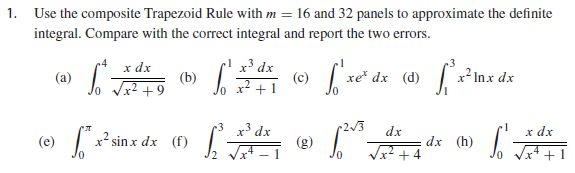# matlab复合梯形法,matlab实现复合梯形法则

function int_f = CompoundEchelon( f, a, b, m )

% input : f : function handler

% a : the lower limit of integral

% b : the upper limit of integral

% m : cut integral area into m peace

% output : int_f : the answer of the integral

h = (b - a) / m;

int_f = 0;

if m >= 2

for i = 1 : m-1

int_f = int_f + 2 * f(a + h * i);

end

end

int_f = int_f + f(a) + f(b);

int_f = int_f * h / 2;

endclear all

format long

clc

%% (a)

fprintf(' (a) \n')

f = @(x) x./((x.^2+9).^0.5);

int1_16 = CompoundEchelon(f, 0, 4, 16);

int1_32 = CompoundEchelon(f, 0, 4, 32);

error1_16 = abs(correct_int1 - int1_16);

error1_32 = abs(correct_int1 - int1_32);

fprintf('int1_16 = %g\n', int1_16);

fprintf('int1_32 = %g\n', int1_32);

fprintf('correct_int1 = %g\n', correct_int1);

fprintf('error1_16 = %g\n', error1_16);

fprintf('error1_32 = %g\n', error1_32);

%% (b)

fprintf(' (b) \n')

f = @(x) (x.^3)./(x.^2+1);

int2_16 = CompoundEchelon(f, 0, 1, 16);

int2_32 = CompoundEchelon(f, 0, 1, 32);

error2_16 = abs(correct_int2 - int2_16);

error2_32 = abs(correct_int2 - int2_32);

fprintf('int2_16 = %g\n', int2_16);

fprintf('int2_32 = %g\n', int2_32);

fprintf('correct_int2 = %g\n', correct_int2);

fprintf('error2_16 = %g\n', error2_16);

fprintf('error2_32 = %g\n', error2_32);

%% (c)

fprintf(' (c) \n')

f = @(x) x.*exp(x);

int3_16 = CompoundEchelon(f, 0, 1, 16);

int3_32 = CompoundEchelon(f, 0, 1, 32);

error3_16 = abs(correct_int3 - int3_16);

error3_32 = abs(correct_int3 - int3_32);

fprintf('int3_16 = %g\n', int3_16);

fprintf('int3_32 = %g\n', int3_32);

fprintf('correct_int3 = %g\n', correct_int3);

fprintf('error3_16 = %g\n', error3_16);

fprintf('error3_32 = %g\n', error3_32);

%% (d)

fprintf(' (d) \n')

f = @(x) (x.^2).*(log(x));

int4_16 = CompoundEchelon(f, 1, 3, 16);

int4_32 = CompoundEchelon(f, 1, 3, 32);

error4_16 = abs(correct_int4 - int4_16);

error4_32 = abs(correct_int4 - int4_32);

fprintf('int4_16 = %g\n', int4_16);

fprintf('int4_32 = %g\n', int4_32);

fprintf('correct_int4 = %g\n', correct_int4);

fprintf('error4_16 = %g\n', error4_16);

fprintf('error4_32 = %g\n', error4_32);

%% (e)

fprintf(' (e) \n')

f = @(x) (x.^2).*(sin(x));

int5_16 = CompoundEchelon(f, 0, pi, 16);

int5_32 = CompoundEchelon(f, 0, pi, 32);

error5_16 = abs(correct_int5 - int5_16);

error5_32 = abs(correct_int5 - int5_32);

fprintf('int5_16 = %g\n', int5_16);

fprintf('int5_32 = %g\n', int5_32);

fprintf('correct_int5 = %g\n', correct_int5);

fprintf('error5_16 = %g\n', error5_16);

fprintf('error5_32 = %g\n', error5_32);

%% (f)

fprintf(' (f) \n')

f = @(x) (x.^3)./((x.^4-1).^0.5);

int6_16 = CompoundEchelon(f, 2, 3, 16);

int6_32 = CompoundEchelon(f, 2, 3, 32);

error6_16 = abs(correct_int6 - int6_16);

error6_32 = abs(correct_int6 - int6_32);

fprintf('int6_16 = %g\n', int6_16);

fprintf('int6_32 = %g\n', int6_32);

fprintf('correct_int6 = %g\n', correct_int6);

fprintf('error6_16 = %g\n', error6_16);

fprintf('error6_32 = %g\n', error6_32);

%% (g)

fprintf(' (g) \n')

f = @(x) 1./((x.^2+4).^0.5);

int7_16 = CompoundEchelon(f, 0, 2*3^0.5, 16);

int7_32 = CompoundEchelon(f, 0, 2*3^0.5, 32);

error7_16 = abs(correct_int7 - int7_16);

error7_32 = abs(correct_int7 - int7_32);

fprintf('int7_16 = %g\n', int7_16);

fprintf('int7_32 = %g\n', int7_32);

fprintf('correct_int7 = %g\n', correct_int7);

fprintf('error7_16 = %g\n', error7_16);

fprintf('error7_32 = %g\n', error7_32);

%% (h)

fprintf(' (h) \n')

f = @(x) x./((x.^4+1).^0.5);

int8_16 = CompoundEchelon(f, 0, 1, 16);

int8_32 = CompoundEchelon(f, 0, 1, 32);

error8_16 = abs(correct_int8 - int8_16);

error8_32 = abs(correct_int8 - int8_32);

fprintf('int8_16 = %g\n', int8_16);

fprintf('int8_32 = %g\n', int8_32);

fprintf('correct_int8 = %g\n', correct_int8);

fprintf('error8_16 = %g\n', error8_16);

fprintf('error8_32 = %g\n', error8_32);

03-17442
03-18

03-23
03-02
11-243746
12-171万+
03-202655
06-15
03-172664
05-205969
05-17661
03-161062
11-043万+
03-172468
11-101万+
06-221万+
01-02690
01-271490

### “相关推荐”对你有帮助么？

•非常没帮助
•没帮助
•一般
•有帮助
•非常有帮助点击重新获取扫码支付余额充值